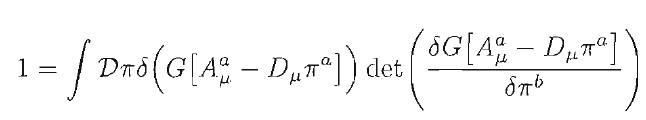In Schwartz QFT book, while explaining the Faddeev-Popov procedure, he presents this following observation at (25.99):Can someone help understanding me why this expression equals one?

THANK YOU!

$$1=\int d^nv \delta^n(\vec v)=\int d^nx\det\left(\frac{\partial v^i}{\partial x^j}\right) \delta^n(\vec v(\vec x))$$
•